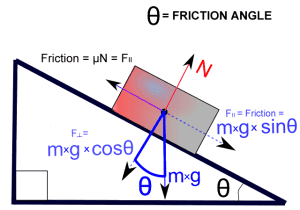# Friction problem involving a block and pulley on a ramp...

## Homework Statement

In the system, find minimum weight of the block 'B' to start the motion of the block 'A'
up the plane.weight of block 'A' 10 KN. The angle of friction for the block and the incline,between the pulley and the string may be assumed as 15° (assume pulley is locked)## Homework Equations

Fr is the resistive force of friction.
μ is the coefficient of friction for the two surfaces (Greek letter "mu")
N is the normal or perpendicular force pushing the two objects together.
μN is μ times N.## The Attempt at a Solution

i don't know how to solve it:([/B]

The problem requires the "minimum" condition, so your job is to make "A" move forwards. Try to analyse the forces on A. If the net force is not 0, then A will start to move.

haruspex